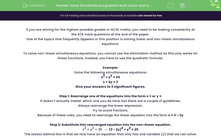# Solve Simultaneous Equations both Linear and Quadratic

In this worksheet, students will practise solving linear, circle and quadratic simultaneous equations.Key stage:  KS 4

Year:  GCSE

GCSE Subjects:   Maths

GCSE Boards:   AQA, Eduqas, OCR, Pearson Edexcel,

Curriculum topic:   Algebra

Curriculum subtopic:   Solving Equations and Inequalities Algebraic Equations

Difficulty level:#### Worksheet Overview

If you are aiming for the highest possible grades in GCSE maths, you need to be looking consistently at the 5/6 mark questions at the end of the paper.

One of the topics that frequently appears in this position is solving linear and non-linear simultaneous equations.

To solve non-linear simultaneous equations, you cannot use the elimination method as this only works for linear functions. Instead, you have to use the quadratic formula.

Example:

Solve the following simultaneous equations:

x2 + y2 = 25

x + 2y = 3

Step 1: Rearrange one of the equations into the form x = or y =

It doesn’t actually matter which one you do here, but there are a couple of guidelines:

Always rearrange the linear expression.

Try to avoid fractions.

Because of these rules, you need to rearrange the linear equation into the form x = 3 – 2y

Step 2: Substitute this rearranged equation into the non-linear equation.

x2 + y2 = 25  →  (3 - 2y)2 + y2 = 25

The reason behind this is that we now have an equation that only has one variable (y) that we can solve.

Step 3: Expand and rearrange.

We can see that the equation (3 - 2y)2 + y2 = 25 is a quadratic, so we need to rearrange it into the form ay2 + by + c = 0

(3 - 2y)2 + y2 = 25

(3 – 2y)(3 – 2y) + y2 = 25

9 – 12y + 4y2 + y2 = 25

5y2 – 12y + 9 = 25

5y2 – 12y - 16 = 0

Step 4: Solve the quadratic to find the values of y.

Sometimes we can factorise this but for this example, you are asked to give your answers to 3 significant figures. This means you have to use the quadratic formula.Evaluating this gives the values: y1 = 3.35 and y2 = -0.954

Step 5: Use the value of y to find the values of x.

All we have to do to find the values of x is to substitute our values of y into the rearranged linear equation.

x1 = 3 – 2(y1) = 3 – 2(3.35) = -3.71

x2 = 3 – 2(y2) = 3 – 2(-0.954) = 4.91

So the solutions to our two simultaneous equations are (-3.71,3.35) and (4.91,-0.954)

Time for some questions.

### What is EdPlace?

We're your National Curriculum aligned online education content provider helping each child succeed in English, maths and science from year 1 to GCSE. With an EdPlace account you’ll be able to track and measure progress, helping each child achieve their best. We build confidence and attainment by personalising each child’s learning at a level that suits them.

Get started